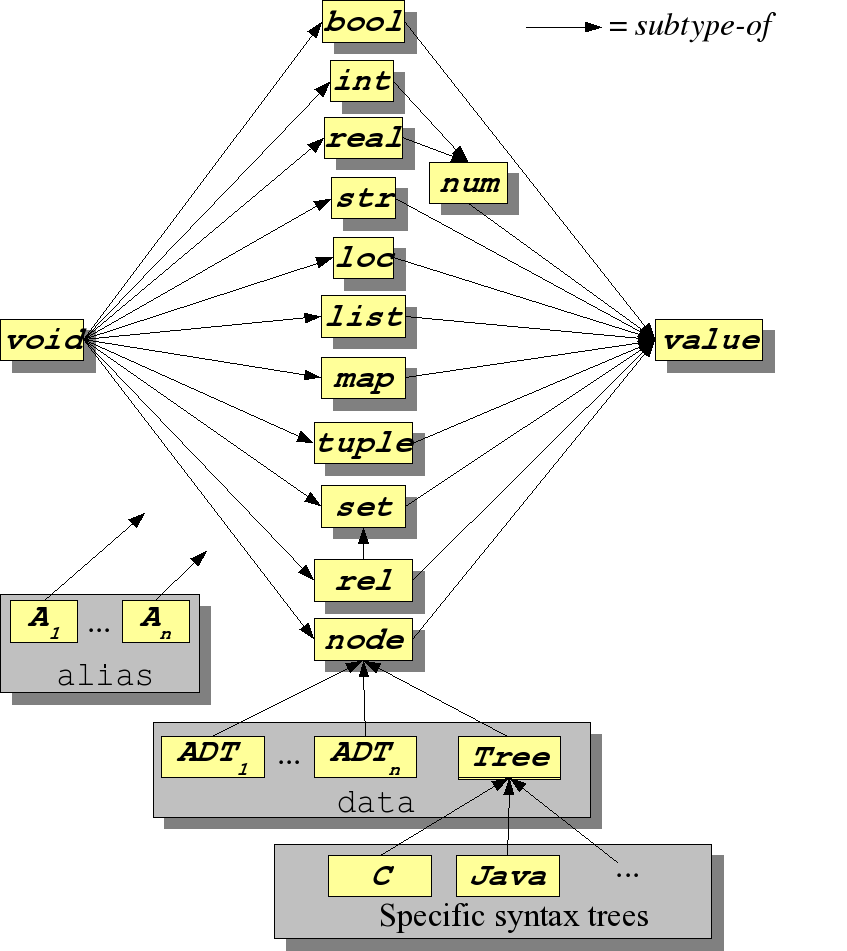# StaticTyping

rascal-0.33.7

#### Synopsis​

The static type system of Rascal.

#### Description​

Rascal is based on static typing, this means that as many errors and inconsistencies as possible are spotted before the program is executed.

## The Type Lattice​

The types are ordered in a so-called type lattice shown in the following figure.The arrows describe a subtype-of relation between types. The type `void` is the smallest type and is included in all other types and the type `value` is the largest type that includes all other types. We also see that `rel` is a subtype of `set` and that each ADT is a subtype of `node`. A special role is played by the datatype `Tree` that is the generic type of syntax trees. Syntax trees for specific languages are all subtypes of `Tree`. As a result, syntax trees can be addressed at two levels:

• in a generic fashion as `Tree` and,
• in a specific fashion as a more precisely typed syntax tree. Finally, each `alias` is structurally equivalent to one or more specific other types.

The fact that the types are ordered in a lattice makes it possible to define a Least Upper Bound (lub) on types. Given two types T₁ and T₂, `lub(T₁, T₂)` is defined as the nearest common super type of T₁ and T₂ in the type lattice.

The Rascal type system has various advanced features that are described separately:

• Types may be be parameterized resulting in very general and reusable types, see Type Parameters.
• Declarations of Functions and Algebraic Data Types may be parameterized and Type Constraints can be used to define constraints on the actual type to be used.
• The formal arguments of functions are bound to values but in exceptional cases a function may need a type as argument value, ReifiedTypes make this possible.

#### Examples​

Here are some simple examples of correct and incorrect typing:

We can assign an integer value to an integer variable:

``rascal>int i = 3;int: 3``

But assigning a string value gives an error:

``rascal>int j = "abc";|prompt:///|(4,9,<1,4>,<1,13>): Expected int, but got strAdvice: |https://www.rascal-mpl.org/docs/Rascal/Errors/CompileTimeErrors/UnexpectedType|ok``

The `num` type accepts integer and real values:

``rascal>num n = i;num: 3rascal>n = 3.14;num: 3.14``

A variable of type `value` accepts all possible values:

``rascal>value v = true;value: truerascal>v = "abc";value: "abc"rascal>v = [1, 2, 3];value: [1,2,3]``